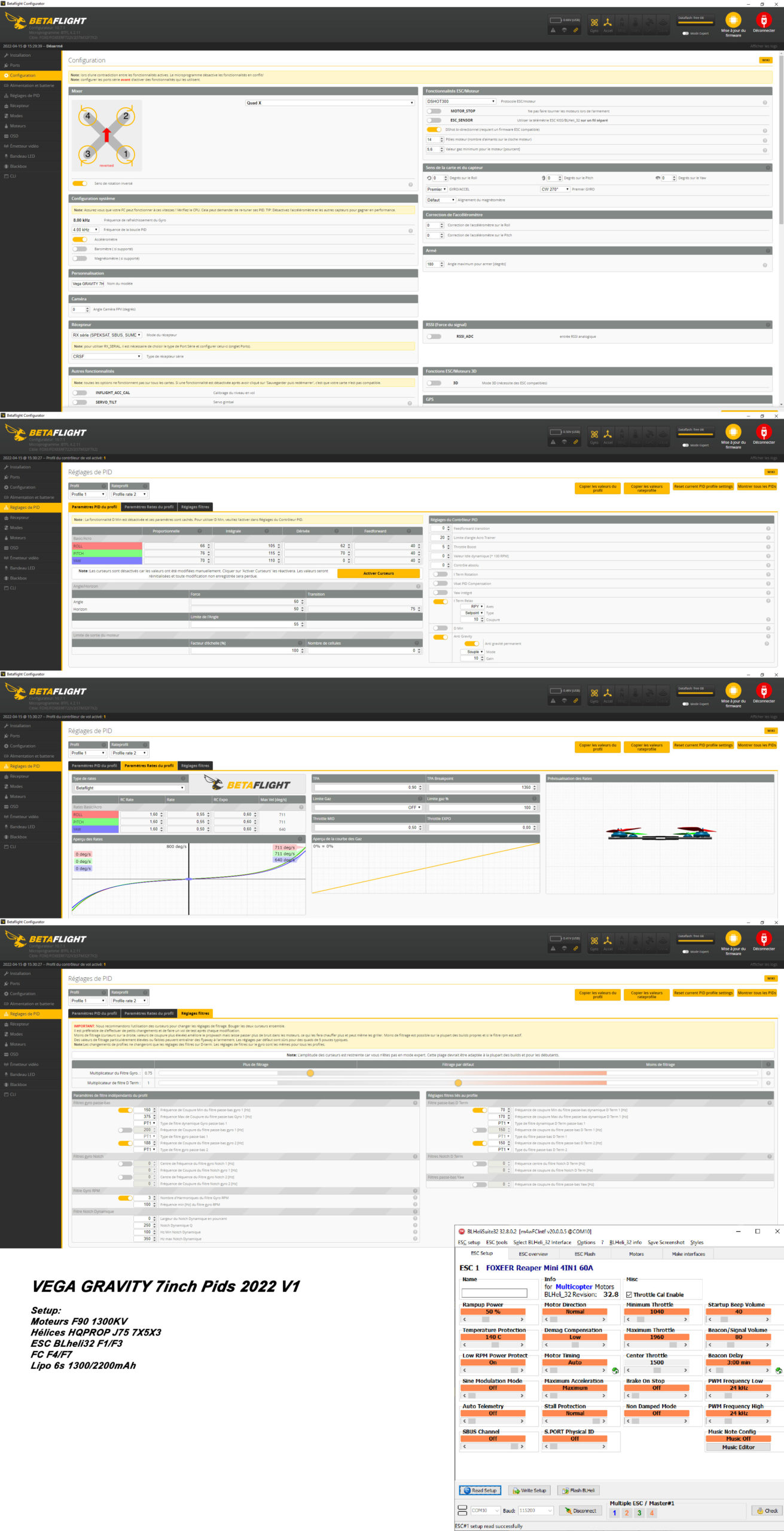﻿ VEGA GRAVITY 7″ 2022 Settings CINEMATIC – PIDs Betaflight 4.2.10 – BlHeli32 32.8+ – Vega FPV Shop www.dauch.fr

# VEGA GRAVITY 7″ 2022 Settings CINEMATIC – PIDs Betaflight 4.2.10 – BlHeli32 32.8+

Réglages 2022 V1 pour le VEGA GRAVITY 7 pouces Betaflight 4.2.10 / BLheli32.

Category:

## Description

Pids & settings 2021 Cinematic for Vega GRAVITY 7″ Frame. Don’t forget to check your motors temperature !

Betaflight Configurator : https://github.com/betaflight/betaflight-configurator/releases

Betaflight Firmware : https://github.com/betaflight/betaflight/releases

Commande CLI :

set gyro_lowpass2_hz = 188
set dyn_notch_width_percent = 0
set dyn_notch_q = 250
set dyn_notch_min_hz = 100
set dyn_notch_max_hz = 350
set dyn_lpf_gyro_min_hz = 150
set dyn_lpf_gyro_max_hz = 375
set acc_calibration = 6,59,-144,1
set rssi_scale = 200
set min_throttle = 1070
set dshot_idle_value = 560
set dshot_bidir = ON
set use_unsynced_pwm = OFF
set motor_pwm_protocol = DSHOT300
set motor_pwm_rate = 480
set yaw_motors_reversed = ON
set small_angle = 180
set pid_process_denom = 2
set thrust_linear = 25
set name = Vega GRAVITY 7HD
set anti_gravity_gain = 10000
set iterm_relax = RPY
set iterm_relax_cutoff = 10
set p_pitch = 76
set i_pitch = 115
set d_pitch = 70
set f_pitch = 40
set p_roll = 66
set i_roll = 105
set d_roll = 62
set f_roll = 40
set p_yaw = 70
set i_yaw = 110
set f_yaw = 40
set d_min_roll = 0
set d_min_pitch = 0
set d_min_boost_gain = 45
set d_min_advance = 30
set roll_rc_rate = 160
set pitch_rc_rate = 160
set yaw_rc_rate = 160
set roll_expo = 60
set pitch_expo = 60
set yaw_expo = 60
set roll_srate = 55
set pitch_srate = 55
set yaw_srate = 50
set tpa_rate = 90
save